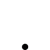# 漲跌成交線語法

•   2.9K
• 最後發表   iakoto  2016 十月 21
iakoto 發文於   2016/10/17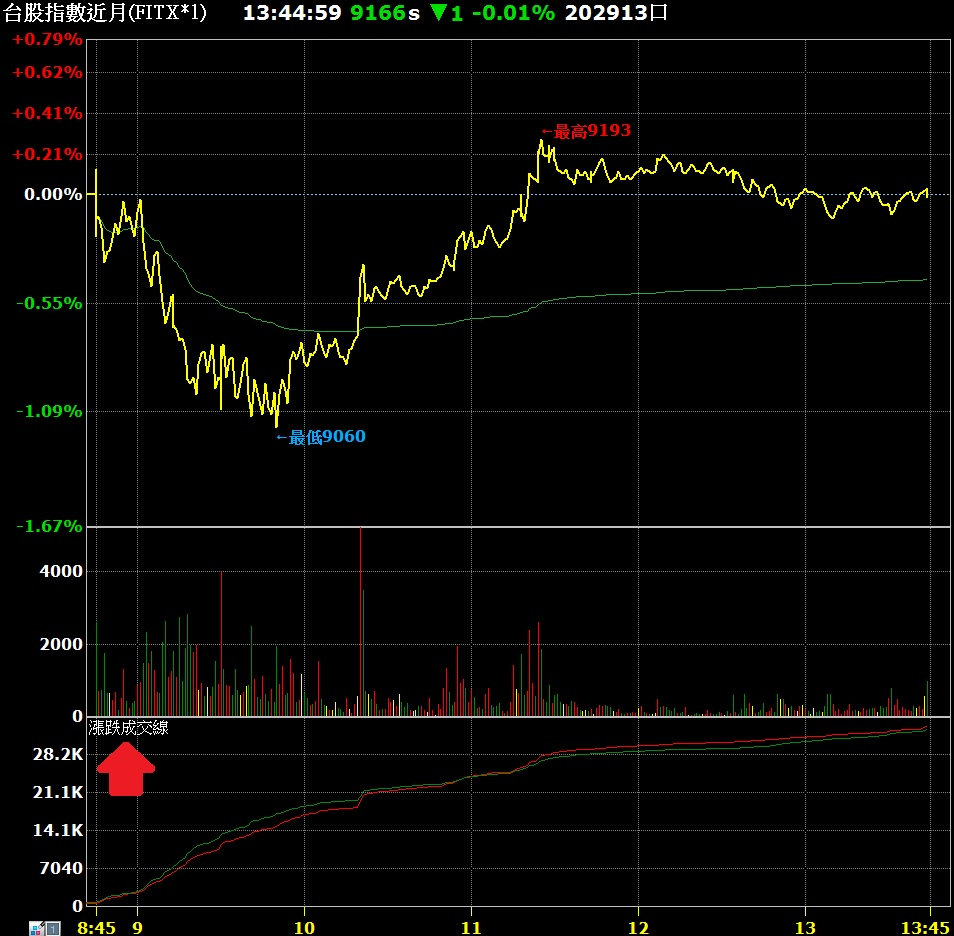XQ小幫手 發文於   2016/10/18

Hi iakoto：

http://www.xq.com.tw/APHelp/DAQBTS/html/functionL.htm

iakoto 發文於   2016/10/18

XQ小幫手 發文於   2016/10/20

Hi 請您試試看以下的程式碼範例，

```// 每一筆K棒內算出這一筆K棒內上漲的tick量/下跌的tick量/平盤的tick量
//
variable: vol_up(0), vol_down(0), vol_even(0);

variable: idx(0);
variable: tick_price(0), tick_preprice(0), tick_vol(0), tick_time(0), tick_date(0);

// 目前只支援分鐘
//
if barfreq <> "Min" then raiseruntimeerror("只支援分鐘");

// Tick資料沒有這麼長, 所以先只算當日的資料
//
if currentdate <> date then return;

//vol_up = 0;
//vol_down = 0;
//vol_even = 0;
idx = 0;

while true
begin
// 判斷這一筆Tick的時間是否還在分鐘的範圍內
//
tick_time = GetField("Time", "Tick")[idx];
tick_date = GetField("Date", "Tick")[idx];
if tick_date <> date or tick_time < time then break;

tick_price = GetField("Close", "Tick")[idx];
tick_preprice = GetField("Close", "Tick")[idx+1];
tick_vol = GetField("Volume", "Tick")[idx];

if tick_price > tick_preprice then vol_up = vol_up + tick_vol;
if tick_price < tick_preprice then vol_down = vol_down + tick_vol;
//if tick_price = tick_preprice then vol_even = vol_even + tick_vol;

idx = idx + 1;
end;

plot1(vol_up);
plot2(vol_down);
//plot3(vol_even);```

iakoto 發文於   2016/10/20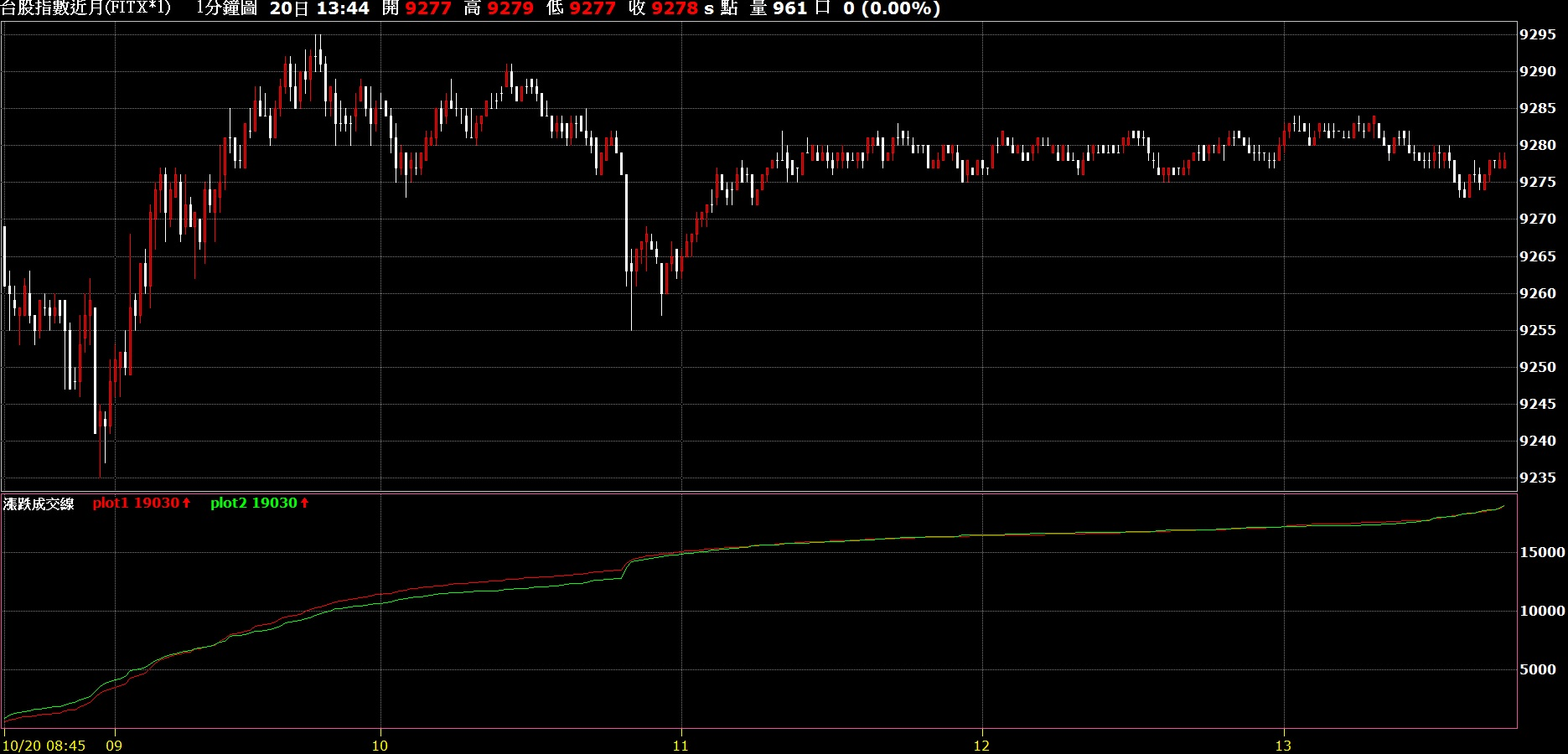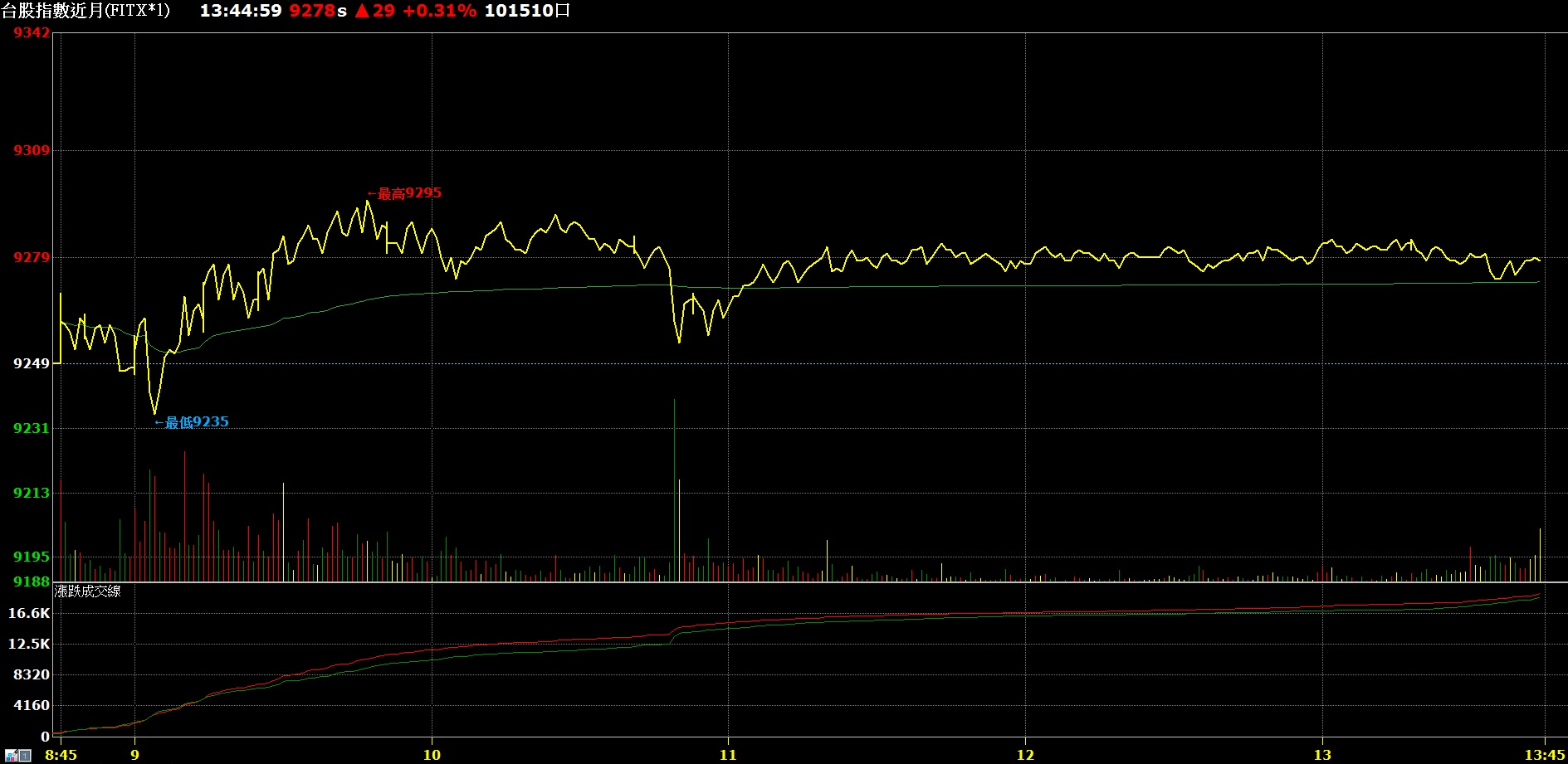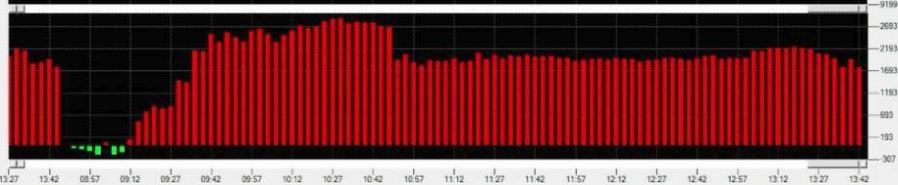XQ小幫手 發文於   2016/10/21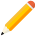發表新主題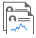我的發文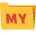我的收藏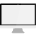分類

Close